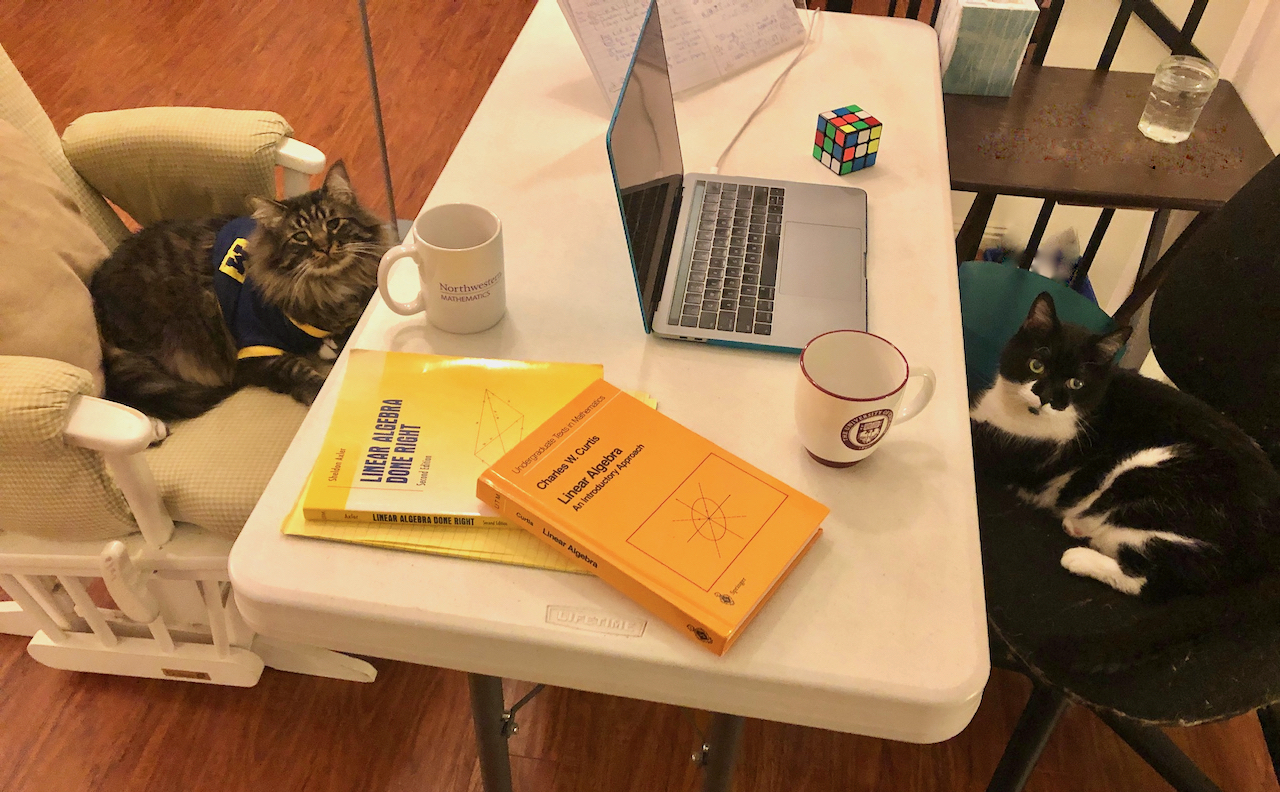## Teaching:

### As an assistant professor of teaching at University of California, Riverside, I have designed, coordinated, and taught the two-quarter introduction to functions (precalculus) sequence Math 6A/6B. I am also developing a hybrid and online version of these courses with my ILTI grant. At UCR I have also taught Math 10A: Multivariate Differential Calculus and Math 131: Linear Algebra 1 (a proof based course). As a post doc at Northwestern University, I taught Math 234 (Multiple Integrals & Vector Calculus), Math 230 (Differential Calculus of Multivariable Functions), Math 220 (Differential Calculus in One Variable) and Math 202 (Finite Mathematics). As a graduate student at the University of Michigan, I taught: Pre-Calculus and Calculus I, II, & III. As an undergraduate student at the University of Chicago, I was a counselor for the Young Scholar's Program, which involved running math discussion sessions for advanced middle and high school students, as well as a Harper mathematics tutor.